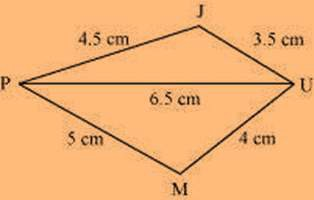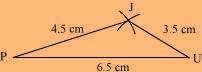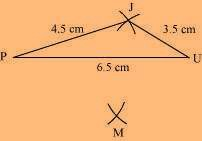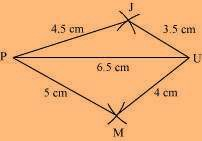# Construct the following quadrilateral: Quadrilateral JUMP. JU = 3.5 cm UM = 4 cm MP = 5 cm PJ = 4.5 cm PU = 6.5 cm

Solution

The rough sketch of the quadrilateral JUMP can be drawn as follows.(1) ∆ JUP can be constructed by using the given measurements as follows.(2) Vertex M is 5 cm away from vertex P and 4 cm away from vertex U.

Considering P and U as centres, draw arcs of radii 5 cm and 4 cm respectively.

Let the point of intersection be M.(3) Join M to P and U.